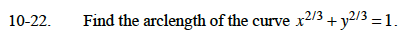### Home > CALC > Chapter 10 > Lesson 10.1.3 > Problem10-22

10-22.The general formula for arc length is:

Rewrite the original equation in y = form.

$y=(1-x^{2/3})^{3/2}$

$y^\prime=\frac{3}{2}(1-x^{2/3})^{1/2}\big(-\frac{2}{3}x^{-1/3}\big)$

Before computing the arclength, graph this curve to determine the bounds of integration and use the symmetry of the graph.